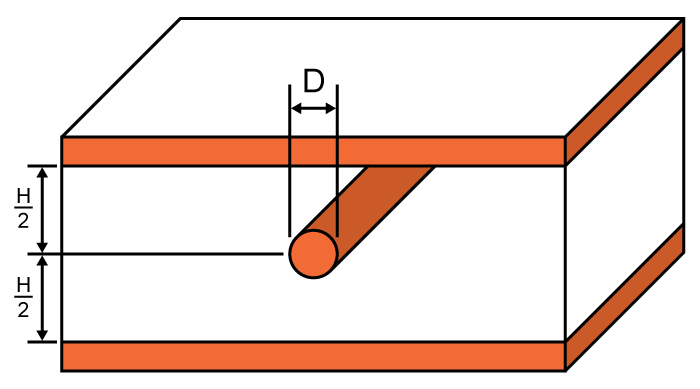# Wire Stripline Impedance Calculator

## A calculator for computing the impedance of a wire stripline.

Ohms

### Overview

A wire stripline is constructed with a circular conductor embedded between two ground planes, with the conductor and ground planes separated by a dielectric. This tool is designed to calculate the characteristic impedance of a wire stripline given the diameter of the wire (conductor) and the height of the substrate (or the distance between the top and bottom ground planes).

To use this tool, just enter the values for the diameter of the wire and the distance between ground planes. It is possible to select the unit of measurement for the wire diameter and the distance between planes. A value of 4 is a good approximation for the dielectric constant if the wire stripline is to be constructed on a printed circuit board.### Equation

$$Z_{0_{ws}}= \frac{\eta _{o}}{2\pi \sqrt{\epsilon_{r}}}\cdot \ln \left ( \frac{4H}{\pi D} \right )$$

Where:

$$Z_{0_{ws}}$$ = characteristic impedance of a wire stripline in ohms (Ω)

$$D$$ = wire diameter

$$H$$ = distance between two ground planes

$$\epsilon_{r}$$ = relative permittivity of the dielectric between the plane(s) and the wire

$$\eta _{o}$$ = wave impedance of free space ≈ 377 Ω

Note: The above equation is from IPC-2141A "Design Guide for High-Speed Controlled Impedance Circuit Boards" and is accurate to within 1% for D ≤ H/2

### Applications

A wire stripline can be used instead of a standard stripline. Note that the only difference in construction between these two is the shape of the sandwiched conductor: a wire stripline uses a round conductor while a standard stripline has a rectangular conductor. As such, both the wire stripline and the standard stripline share common characteristics.

Striplines are more immune to noise brought by radio frequency signals because the conductor is "hidden" between two ground planes. However, this is at the expense of slower propagation speeds because striplines have a higher relative permittivity. Note that the propagation speed of signals is inversely proportional to the effective permittivity (the higher the permittivity, the slower the signal travels through a material). Striplines, especially wire striplines, are also much more difficult to manufacture than microstrips.